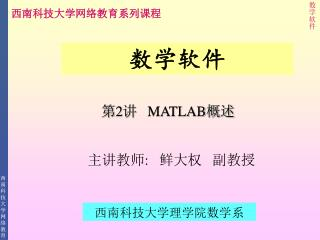DownloadDownload Presentation# 第 2 讲 MATLAB 概述

Download Presentation## 第 2 讲 MATLAB 概述

- - - - - - - - - - - - - - - - - - - - - - - - - - - E N D - - - - - - - - - - - - - - - - - - - - - - - - - - -
##### Presentation Transcript

1. 数学软件 西南科技大学网络教育系列课程 第2讲 MATLAB概述 数学软件 主讲教师: 鲜大权 副教授 西南科技大学理学院数学系

2. 2.1 MATLAB的发展 2.2 MATLAB的主要功能 2.3 MATLAB系统的运行环境与安装 2.4 MATLAB系统的启动与退出 2.5 MATLAB命令窗口 2.6 MATLAB文件管理 2.7 MATLAB帮助系统 2.8 MATLAB功能演示

3. 2.1 MATLAB的发展 • 1980年前后，MATLAB的雏形：LINPACK 和EISPACK的接口程序。 • 1984年成立MathWorks公司，推出MATLAB第1版(DOS版)。 • 1992年推出4.0版。 • 1994年推出4.2版。 • 1997年推出5.0版。 • 1999年初推出5.3版。 • 2001年7月，推出最新版本6.1版。

4. 2.2 MATLAB的主要功能 • 1. 数值计算和符号计算功能 • 2. 绘图功能 • 3. MATLAB语言体系 • 4. MATLAB工具箱

5. 2.3 MATLAB的运行环境与安装 • 2.3.1 运行环境 • 1. 硬件环境 • 2. 软件环境 • 2.3.2 安装 • (1)启动Windows 98/XP。 • (2)将MATLAB 6.5光盘放入光驱，运行setup.exe文件。 • (3)按界面提示进行操作。

6. 2.4 MATLAB系统的启动与退出 • 2.4.1 启动 • 有3种常见方法： • (1) “开始”“程序”“Matlab”“MATLAB 6.5” 。 • (2)运行MATLAB系统启动程序matlab.exe。 • (3)建立MATLAB快捷方式。

7. 2.4.2 退出 • 也有3种常见方法： • (1)在MATLAB命令窗口File菜单中选择Exit MATLAB命令。 • (2)在MATLAB命令窗口输入Exit或Quit命令。 • (3)单击MATLAB命令窗口的关闭按钮。

8. 2.5 MATLAB命令窗口 • 2.5.1 菜单栏 • 在MATLAB 6.5命令窗口的菜单栏，共包含File、Edit、View、Window和Help等5个菜单项。 • 2.5.2 工具栏 • MATLAB 6.53命令窗口的工具栏共提供了10个命令按钮。

9. 2.5.3 命令编辑区 • 1. 命令编辑区的作用 • 命令编辑区用于输入命令和显示计算结果。 • 2. 命令行的输入规则 一个命令行输入一条命令，命令行以回车结束。 一个命令行也可以输入若干条命令，各命令之间以逗号分隔，若前一命令后带有分号，则逗号可以省略。 如果一个命令行很长，要加续行符（三个小黑点…）。 • 3. 命令行的编辑 • 4. 常用操作系统命令

10. 2.6 MATLAB文件管理 • 2.6.1 MATLAB的目录结构 • 2.6.2 MATLAB的搜索路径 • 2.6.3 用户目录的设置 • 将用户目录设置成当前目录使用cd命令。 • 注意：设置的当前目录只是在当前启动的MATLAB环境下有效，一旦MATLAB重新启动，必须重新设置。

11. 2.6.4 搜索路径的设置 • 1. 用path命令设置搜索路径 • 使用path命令可以把用户目录临时纳入搜索路径。 • 注意: 设置的搜索路径仅在当前启动的MATLAB环境下有效，一旦MATLAB重新启动，必须重新设置。 • 2. 用路径浏览器设置搜索路径 • 路径浏览器可用来设置当前目录和永久性改变搜索路径。 • (1)路径浏览器的启动 • (2)路径浏览器的操作

12. 2.7 MATLAB帮助系统 • 2.7.1 帮助命令 • 1. help命令 • help命令是查询函数语法的最基本方法，查询信息直接显示在命令窗口。 • 2. lookfor命令 • lookfor对搜索范围内的M文件进行关键字搜索，条件比较宽松。 • 2.7.2 帮助窗口 • 2.7.3 帮助桌面 • 2.7.4 在线帮助页

13. 还可以访问MathWorks公司的主页(http://www.mathworks.com):还可以访问MathWorks公司的主页(http://www.mathworks.com):

14. 2.8 MATLAB功能演示 • 例2.1 求解线性方程组 • 命令如下： • a=[2,3,-1;8,2,3;45,3,9]; • b=[2;4;23]; • x=inv(a)*b

15. 例2.2 绘制正弦曲线和余弦曲线。 • 命令如下： • x=[0:0.5:360]*pi/180; • plot(x,sin(x),x,cos(x)) ; • 例2.3 输入10个学生的成绩并对成绩按升序排序。 • 在MATLAB命令窗口输入： • g=input('请输入学生成绩:'); • g=sort(g)

16. 例2.4 设有常微分方程初值问题，试求其数值解，并与精确解相比较。 • (1)建立函数文件funt.m： • function yp=funt(t,y) • yp=(y^2-t-2)/4/(t+1); • (2)求解微分方程： • t0=0;tf=10;y0=2; • [t,y]=ode23('funt',[t0,tf],y0); • y1=sqrt(t+1)+1; • t'